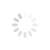# Excel VBA Basics - Express Guide & Ready-To-Go Code

## All you need to know to get rid of 80% of your routine and focus on more interesting tasks

In the first part we will discuss instruments, which I wish I have known how to use when I have started my first job as a Budget Analyst. They would have allowed me to automate 80% of the routine and save hundreds of hours of time.

This article is written for those, who are stuck at the lesson Excel-VBA 101 - variables and types. I have been there myself. These tutorials didn't really work for me because before actually doing anything you have to pass through an hour or two listening about some variables, types and doing stupid stuff like printing(1+1*1). So here we will skip all that and focus on things that really get our job done. Yes, this is insufficient to become a real programmer, but in my opinion all other stuff starts to matter when as soon as you start writing complex scripts, so I propose to leave the boring technical stuff for the later.

This website is my toolbox which I share with everyone, so I recommend not to waste your time to type any of the code, just copy and paste it (you are not at school, let's get the job done :). It will take just 10 minutes to go through, so activate macros in your Excel, press ALT+F11 and type let's start:

### How to address Cells in a Workbook

Let's skip all the boring part about the Excel object model for now and just focus on one particular object which you will allow you to do 80% of the job with workbooks - workbook cells. As noobs we are going to use the safest way to address a cell in a workbook, so that by accident we won't do damage to other workbooks/worksheets. For the safety reasons we will write our macros only in workbook modules for now.Now that we have created a module we open it and start coding. This is how we address an Excel cell:

``````'Thisworkbook.Sheets("SheetName").Cells(1,1).value - how we address a cell
'To write a comment you have to use ' in front of the line

x=1 ' variable

'How to change the value of cell A1 to the value equal to x
Thisworkbook.Sheets("SheetName").Cells(1,1).Value = x

'we read the value of cell A1 of the sheet "SheetName" in a variable x
x = Thisworkbook.Sheets("SheetName").Cells(1,1).Value ``````

If you understood what is what in this code then just skip this paragraph and move on to the next part. If not, here is explanation:

ThisWorkbook is the workbook the macro is written in (that's the reason why we want to write our code inside of our workbook, so that we are sure we don't address any other element in another Workbook and destroy valuable data in it.

Sheets("SheetName") addresses to a sheet called "SheetName" in ThisWorkbook.

Cells(1,1).value - addresses a value in a cell (row, column).

Now that we know how to address a cell in a Workbook let's try to do something.

How will we run a macro? Simplest way - to create a shape and assign macro to it, so that next time we want to run our script we just have to click the rectangle.### Cycles

FOR cycle is the second must-know in Excel-VBA. This is essential for making Excel do stuff repeatedly.

``````Sub MyFirstSub ()

'Fill the first 100 cells in a column with values equal to RowNumber*3
for RowNumber = 1 to 100
Thisworkbook.Sheets("Sheet1").Cells(RowNumber,1).value = RowNumber*3
next RowNumber

End Sub``````

Yes, this is not too cool yet, but wait. we are getting there.

### Arrays

Arrays allow us to create a loopable variables.

``````Sub MySecondSub ()

' we create an array.
' This particular type of array can store both numbers and strings
' so we don't have to care about types for now

Dim x(100) as Variant

'Read values of 100 cells of the first column of the sheet "Sheet1"
for i = 1 to 100

x(i) = Thisworkbook.Sheets("Sheet1").Cells(i,1)

next i

'Do some stuff with it in memory (for example - divide by 2)
for i = 1 to 100

x(i) = x(i)/2

next i

'Write the result in column 2 of the same worksheet
for i = 1 to 100

Thisworkbook.Sheets("Sheet1").Cells(i,1) = x(i)

next i

End Sub``````

### Conditions and LIKE operator

Now that's where it gets cool. Let's introduce logic to what we are doing. Now let's fill first 10 cells of the workbook with random text. Now let this code copy only those cells, which contain letter "x" in them:

``````Sub MyThirdSub()

' we create an array.
' This particular type of array can store both numbers and strings
' so we don't have to care about types for now

Dim x(10) As Variant

'Read values of 10 cells of the first column of the sheet "Sheet1"
For i = 1 To 10

x(i) = ThisWorkbook.Sheets("Sheet1").Cells(i, 1)

Next i

'Do some stuff with it in memory
For i = 1 To 10

If Not x(i) Like "*" & "x" & "*" Then
x(i) = ""
End If

Next i

'Write the result in column 2 of the same worksheet
For i = 1 To 10

ThisWorkbook.Sheets("Sheet1").Cells(i, 2) = x(i)

Next i

End Sub
``````

Result:(Do not download files from any suspicious websites. It is always safer to copy-paste code in your own files (assuming that you know what the code does of course)).